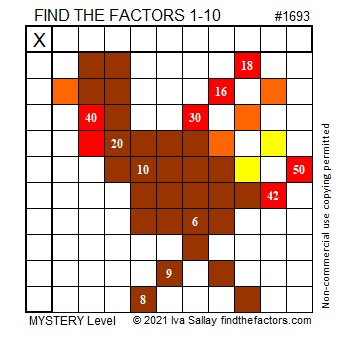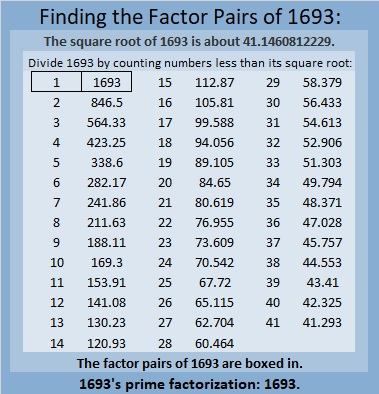# 1693 Tricky Turkey

Contents

### Today’s Puzzle:

You cannot gobble this turkey up unless you can find all of its factors!

Use logic and multiplication facts. It won’t be easy, but write the numbers from 1 to 10 in both the first column and the top row so that those numbers and the given clues make a multiplication table.Here’s the same puzzle without the added color:### Factors of 1693:

• 1693 is a prime number.
• Prime factorization: 1693 is prime.
• 1693 has no exponents greater than 1 in its prime factorization, so √1693 cannot be simplified.
• The exponent in the prime factorization is 1. Adding one to that exponent we get (1 + 1) = 2. Therefore 1693 has exactly 2 factors.
• The factors of 1693 are outlined with their factor pair partners in the graphic below.

How do we know that 1693 is a prime number? If 1693 were not a prime number, then it would be divisible by at least one prime number less than or equal to √1693. Since 1693 cannot be divided evenly by 2, 3, 5, 7, 11, 13, 17, 19, 23, 29, 31, 37, or 41, we know that 1693 is a prime number.### More About the Number 1693:

1693 is the sum of two squares:
37² + 18² = 1693.

1693 is the hypotenuse of a Pythagorean triple:
1045-1332-1693, calculated from 37² – 18², 2(37)(18), 37² + 18².

Here’s another way we know that 1693 is a prime number: Since its last two digits divided by 4 leave a remainder of 1, and 37² + 18² = 1693 with 37 and 18 having no common prime factors, 1693 will be prime unless it is divisible by a prime number Pythagorean triple hypotenuse less than or equal to √1693. Since 1693 is not divisible by 5, 13, 17, 29, 37, or 41, we know that 1693 is a prime number.

## 2 thoughts on “1693 Tricky Turkey”

1.Duncan

Just a quick observation. All prime numbers are the odd leg of primitive Pythagorean triples (ppt) but only a subset can be both the odd leg or the hypotenuse of another ppt. For example (3,4,5), (5,12,13), (13,84,85). The subset of the prime numbers that have this duality are of the form1 mod 12, or 5 mod 12. Thus if a prime number is the hypotenuse of a ppt then it is also the odd leg of another ppt.

•ivasallay

I didn’t realize that the prime numbers with that duality were of the form1 mod 12, or 5 mod 12. Thank you for letting me know!

This site uses Akismet to reduce spam. Learn how your comment data is processed.